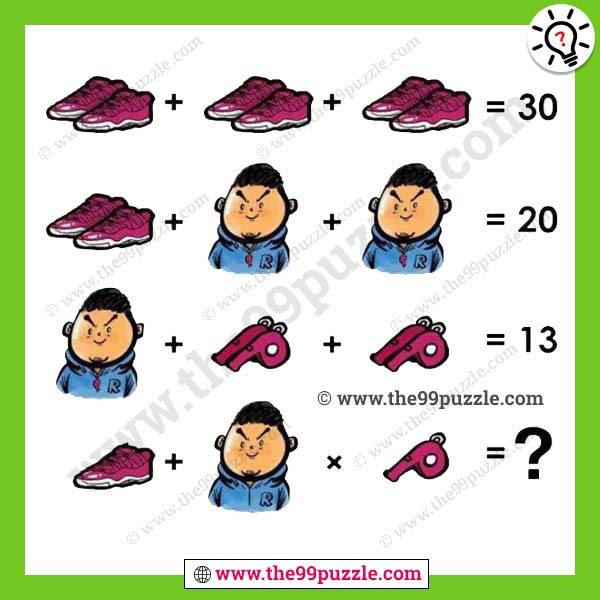# Shoe man whistle puzzle for genius – Puzz104

Shoe man whistle puzzle with the solution. Solve the tricky picture puzzles. In this Math Picture Puzzle, you are given some algebraic equations. Solve these math equations, to find the values of the individual and then solve the last equation to find the value of the missing number which will replace the question mark.###### Explanation:

Single shoe = 5

Man = 3

Single whistle = 2

10+10+10=30

10+5+5=20

5+(2+2)+(2+2)=13

5+3×2=11